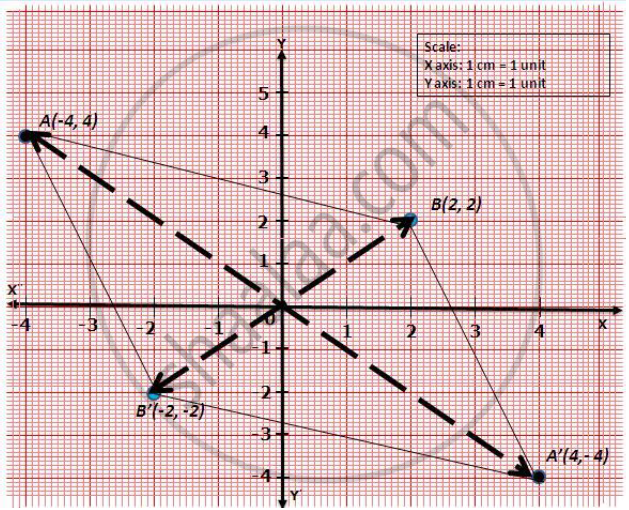# Using Graph Paper and Taking 1 Cm = 1 Unit Along Both X-axis and Y-axis. 1) Plot the Points A(–4, 4) and B(2, 2). 2) Reflect a and B in the Origin to Get the Images A’ and B’ Respectively. 3) Write Down the Coordinates of A’ and B’. 4) Give the Geometrical Name for the Figure Aba’B’. 5) Draw and Name Its Lines of Symmetry. - Mathematics

Using graph paper and taking 1 cm = 1 unit along both x-axis and y-axis.

1) Plot the points A(–4, 4) and B(2, 2).

2) Reflect A and B in the origin to get the images A’ and B’ respectively.

3) Write down the coordinates of A’ and B’.

4) Give the geometrical name for the figure ABA’B’.

5) Draw and name its lines of symmetry.

#### Solution

Taking 1 cm = 1 unit on graph paper, the points are plotted as below:

(1) and (2)(3) The image of A' = (4, –4) and B' = (–2, –2)

(4) Now, AB, A'B, AB' and A'B' are equal in length. So, ABA'B' is a rhombus.

(5) The lines of symmetry are the diagonals of the rhombus ABA'B', i.e. AA' and BB'.

Concept: Concept of Lines Symmetry
Is there an error in this question or solution?